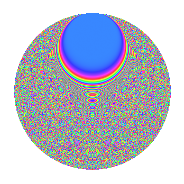# Properties

 Label 1350.2.bfLevel 1350 Weight 2 Character orbit bf Rep. character $$\chi_{1350}(79,\cdot)$$ Character field $$\Q(\zeta_{90})$$ Dimension 2160 Sturm bound 540

# Related objects

## Defining parameters

 Level: $$N$$ = $$1350 = 2 \cdot 3^{3} \cdot 5^{2}$$ Weight: $$k$$ = $$2$$ Character orbit: $$[\chi]$$ = 1350.bf (of order $$90$$ and degree $$24$$) Character conductor: $$\operatorname{cond}(\chi)$$ = $$675$$ Character field: $$\Q(\zeta_{90})$$ Sturm bound: $$540$$

## Dimensions

The following table gives the dimensions of various subspaces of $$M_{2}(1350, [\chi])$$.

Total New Old
Modular forms 6576 2160 4416
Cusp forms 6384 2160 4224
Eisenstein series 192 0 192

## Trace form

 $$2160q + 24q^{5} + O(q^{10})$$ $$2160q + 24q^{5} + 42q^{15} - 12q^{20} + 72q^{21} - 72q^{25} - 288q^{26} - 36q^{30} - 36q^{31} - 18q^{35} + 24q^{36} + 60q^{39} + 12q^{44} - 114q^{45} + 150q^{47} + 30q^{48} + 12q^{50} + 36q^{54} - 18q^{59} - 18q^{60} - 270q^{64} + 6q^{65} - 180q^{67} - 12q^{75} + 480q^{77} + 36q^{81} + 150q^{87} + 132q^{89} - 30q^{90} - 120q^{92} + 18q^{95} + 60q^{99} + O(q^{100})$$

## Decomposition of $$S_{2}^{\mathrm{new}}(1350, [\chi])$$ into newform subspaces

The newforms in this space have not yet been added to the LMFDB.

## Decomposition of $$S_{2}^{\mathrm{old}}(1350, [\chi])$$ into lower level spaces

$$S_{2}^{\mathrm{old}}(1350, [\chi]) \cong$$ $$S_{2}^{\mathrm{new}}(675, [\chi])$$$$^{\oplus 2}$$

## Hecke Characteristic Polynomials

There are no characteristic polynomials of Hecke operators in the database